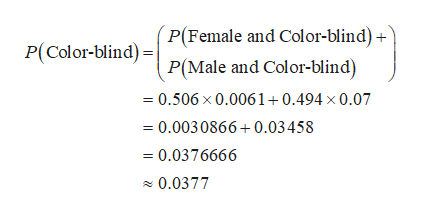# In a given population approximately 7% of all males are color-blind, while only about 0.61 % of women are color-blind. Six years ago 49.4% of the population were male and 50.6% were female.(a) What is the probability that a randomly selected person is color-blind?(b) What is the probability that a randomly selected person who is color-blind is female?(a) The probability that a randomly selected person from the population is color-blind is approximately(Do not round until the final answer. Then round to four decimal places as needed.)(b) The probability that a randomly selected person from the population who is color-blind is female is approximately(Use the answer from part (a) to find this answer. Do not round until the final answer. Then round to four decimal places as needed.)Enter your answer in each of the answer boxes.547 PM10/23/2019Type here to searchSUSsc765432UYTEWK

Question
1 views

HELPhelp_outlineImage TranscriptioncloseIn a given population approximately 7% of all males are color-blind, while only about 0.61 % of women are color-blind. Six years ago 49.4% of the population were male and 50.6% were female. (a) What is the probability that a randomly selected person is color-blind? (b) What is the probability that a randomly selected person who is color-blind is female? (a) The probability that a randomly selected person from the population is color-blind is approximately (Do not round until the final answer. Then round to four decimal places as needed.) (b) The probability that a randomly selected person from the population who is color-blind is female is approximately (Use the answer from part (a) to find this answer. Do not round until the final answer. Then round to four decimal places as needed.) Enter your answer in each of the answer boxes. 547 PM 10/23/2019 Type here to search SUS sc 7 6 5 4 3 2 U Y T E W K fullscreen
check_circle

Step 1

It is given that percentage of males are color-blind is 7%.

The percentage of females are color-blind is 0.61%.

Six years ago, the percentage of population were male is 49.4% and female is 50.6%.

Step 2

a)

The probability that a randomly selected person from the ...help_outlineImage TranscriptioncloseP(Female and Col or-blind)- P(Male and Color-blind) P(Color-blind)= 0.506 x 0.0061+0.494 x 0.07 0.0030866 0.03458 0.0376666 0.0377 fullscreen

### Want to see the full answer?

See Solution

#### Want to see this answer and more?

Solutions are written by subject experts who are available 24/7. Questions are typically answered within 1 hour.*

See Solution
*Response times may vary by subject and question.
Tagged in

### Statistics